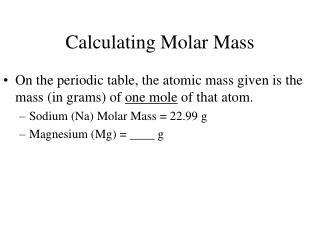DownloadDownload PresentationCalculating Molar Mass

# Calculating Molar Mass

Télécharger la présentation## Calculating Molar Mass

- - - - - - - - - - - - - - - - - - - - - - - - - - - E N D - - - - - - - - - - - - - - - - - - - - - - - - - - -
##### Presentation Transcript

1. Calculating Molar Mass • On the periodic table, the atomic mass given is the mass (in grams) of one mole of that atom. • Sodium (Na) Molar Mass = 22.99 g • Magnesium (Mg) = ____ g

2. What about molecules? • NaCl • has one of each atom • = 22.990 + 35.453 = 58.443 g in one mole of NaCl • H20 • has 2 hydrogen and 1 oxygen • = 2 (1.0079) + 15.999= 18.0148 g in one mole of H20

3. You need to be very careful when counting the number of atoms of each element in a molecule. • Count the number of atoms for each element in the molecule: • Ca(OH)2 • Na2(CO3) • CH3OH

4. Find the molar mass • NH3 • CO3 • HCl • Mg(OH)2 • Fe3(PO4)2

5. Converting moles to mass • Determine the molar mass of one mole of that molecule • Use a conversion factor • 1 mole = ____ g (molar mass)

6. Determine the number of moles: • 25 g Li • 251 g F2

7. Determine the mass: • 2.63 moles of CuSO4 • 0.73 moles of Mg(OH)2

8. Volume • Applies to gases ONLY at STP: 1 mole = 22.4 L • This is referred to as molar volume • STP- Standard Temperature (0°) & Pressure (1 atm)

9. How many grams are there in 28.6 L of carbon monoxide?